# Current Electricity Chapter 3 Extra Questions for Class 12 Physics## myCBSEguide App

Complete Guide for CBSE Students

NCERT Solutions, NCERT Exemplars, Revison Notes, Free Videos, CBSE Papers, MCQ Tests & more.

Current Electricity Chapter 3 Extra Questions for Class 12 Physics. We know Physics is tough subject within the consortium of science subjects physics is an important subject. But if you want to make career in these fields like IT Consultant, Lab Technician, Laser Engineer, Optical Engineer etc. You need to have strong fundamentals in physics to crack the exam. myCBSEguide has just released Chapter Wise Question Answers for class 12 Physics. There chapter wise Extra Questions with complete solutions are available for download in myCBSEguide website and mobile app. These Questions with solution are prepared by our team of expert teachers who are teaching grade in CBSE schools for years. There are around 4-5 set of solved Physics Extra Questions from each and every chapter. The students will not miss any concept in these Chapter wise question that are specially designed to tackle Board Exam. We have taken care of every single concept given in CBSE Class 12 Physics syllabus and questions are framed as per the latest marking scheme and blue print issued by CBSE for class 12.

Class 12 Physics Extra Questions

## Chapter 3 Class 12 Physics Important Questions

Class – 12 Physics (Current Electricity)

1. An ammeter together with an unknown resistance in series is connected across two identical batteries each of emf 1.5 V. When the batteries are connected in series, the galvanometer records a current of 1A and when the batteries are in parallel, the current is 0.6A. What is the internal resistance of each battery?

1. {tex}{1 \over 5}\Omega {/tex}
2. {tex}{1 \over 3}\Omega {/tex}
3. {tex}{1 \over 4}\Omega {/tex}
4. {tex}{1 \over 2}\Omega {/tex}
2. The resistance of a metallic conductor increases due to

1. Change in dimensions of the conductor
2. Change in carrier density
3. Increase in the number of collisions between the carriers
4. Increase in the rate of collisions between the carriers and vibrating atoms of the conductor
3. In the figure, when an ideal voltmeter is connected across 4000 ohm resistance, it reads 30 volts. If the voltmeter is connected across 3000 ohms resistance. It will read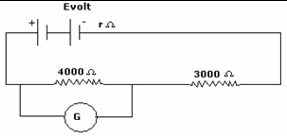1. 22.5 volt
2. 40 volt
3. 35 volt
4. 20 volt
4. The wire of the potentiometer has resistance 4 ohms and length 1 m. It is connected to a cell of e.m.f. 2 volts and internal resistance 1 ohm. The p.d. across the potentiometer wire is:

1. 1.2 V
2. 1.6 V
3. 0.8 V
4. 2.0 V
5. According to Kirchhoff’s Loop Rule

1. The absolute sum of changes in potential around any closed loop must be zero.
2. The algebraic sum of changes in potential around any closed loop must be zero.
3. The algebraic sum of changes in potential around any closed loop must be positive.
4. The algebraic sum of changes in potential around any closed loop must be negative.
6. Plot a graph showing a variation of current versus voltage for the material GaAs.
7. I-V graph for a metallic wire at two different temperatures T1 and T2 is as shown in the figure below. Which of the two temperature is lower and why?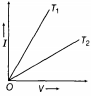8. Two conducting wires X and Y of same diameter but different materials are joined in series across a battery. If the number density of electrons in X is twice than that in Y, then find the ratio of drift velocity of electrons in the two wires.
9. The number density of free electrons in a copper conductor is 8.5 {tex}\times{/tex} 1028 m-3 . How long does an electron take to drift from one end of a wire 3.0 m long to its other end? The area of cross section of the wire is 2.0 {tex}\times{/tex} 10-6m2 and it is carrying a current of 3.0 A.
10. State the reason, why Ga As is most commonly used in making of a solar cell.
11. For the circuit diagram of a Wheatstone bridge shown in the figure, use Kirchhoff’s laws to obtain its balance condition.1. Three resistors {tex}2\Omega ,4\Omega {/tex} and {tex}5\Omega {/tex} are combined in parallel. What is the total resistance of the combination?
2. If the combination is connected to a battery of emf 20 V and negligible internal resistance, determine the current through each resistor, and the total current drawn from the battery?
12. Calculate the value of the resistance R in the circuit shown in the figure, so that the current in the circuit is 0.2A. What would be the potential difference between points A and B?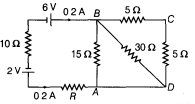13. Calculate the steady current through the {tex}2\Omega{/tex} resistor in the circuit shown in the figure.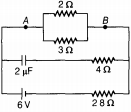14. A wire of resistance {tex}8\Omega{/tex} is bent in the form of a circle. What is the effective resistance between the ends of a diameter AB?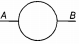Class – 12 Physics (Current Electricity)

1. {tex}{1 \over 3}\Omega {/tex}
Explanation: {tex}E_1=E_2=1.5{/tex}
Let internal resistance of battery be r. If batteries are connected in series then,
{tex}E=E_1+E_2=3\; V{/tex}
Ttotal = 2r
Now,
{tex}I = \frac{E}{{\left( {R + 2r} \right)}}{/tex}
{tex} \Rightarrow 1 = \frac{3}{{\left( {R + 2r} \right)}}{/tex}
R + 2r = 3 ….. (i)
If batteries are connected in parallel
E = 1.5V
{tex}\frac{1}{{{T_{total}}}} = \frac{1}{r} + \frac{1}{r}{/tex}
{tex}{T_{total}} = \frac{r}{2}{/tex}
and,
{tex}0.6 = \frac{{1.5}}{{\left( {R + \frac{r}{2}} \right)}}{/tex}
{tex} \Rightarrow 0.6R + 0.3r = 1.5{/tex} ……. (ii)
on solving equation (i) and (ii)
{tex}r = \frac{1}{3}\Omega {/tex}
1. Increase in the rate of collisions between the carriers and vibrating atoms of the conductor
Explanation: When temperature increases, the thermal speed of the electrons increases as well as, the amplitude of vibration of the positive ions inside the metal conductor also increase, about their mean positions. Thus, the collisions between the electrons and the positive metal ions become more frequent and this decreases the relaxation time, t, leading to an increase in the resistivity of the conductor.
1. 22.5 volt
Explanation: An ideal voltmeter does not draw any current. Therefore the resistances 4000 Ω and 3000 Ω are in series. The current in the circuit
{tex}I = \frac{{{V_{4000}}}}{{4000}} = \frac{{30}}{{4000}}A{/tex}
The potential difference across the 3000 Ω resistor
{tex}{V_{3000}} = I \times 3000{/tex}
{tex} \Rightarrow {V_{3000}} = \frac{3}{{400}} \times 3000{/tex}
{tex}{V_{3000}} = \frac{{90}}{4}{/tex}
{tex}{V_{3000}} = 22.5\;V{/tex}
1. 1.6 V
Explanation: If the battery has an e.m.f E, the resistance of the potentiometer is R and internal resistance of the battery is r, then the current I flowing in the potentiometer wire is given as,
{tex}{I = \frac{E}{{\left( {R + r} \right)}}}{/tex}
{tex}{I = \frac{2}{{\left( {4 + 1} \right)}}}{/tex}
I = 0.4 A
The potential difference V across the potentiometer is
{tex}{V = I \times R}{/tex}
{tex}{ \Rightarrow V = 0.4 \times 4}{/tex}
V = 1.6 V
1. The algebraic sum of changes in potential around any closed loop must be zero.
Explanation: Kirchhoff’s loop rule is based on the principle of conservation of energy. Since work done in transporting a charge in a closed loop is zero. The algebraic sum ( since potential differences can be both positive and negative) of potential differences around any closed loop is always zero.
1. Variation of current versus voltage for the material Ga-As semiconductor material can be shown as –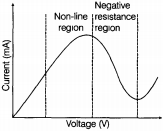It shows at first current increases with applied voltage and then decreases in non linear fashion.
2. To solve this problem we will use ohm’s law which states that V = I {tex}\times{/tex} R
1/R = I/V
So slope of the graph is inversely proportional to resistance.Consider the figure,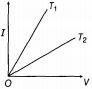Since, slope of 1 > slope of 2.
{tex}I _ { 1 } / V _ { 1 } > I _ { 2 } / V _ { 2 } \Rightarrow V _ { 2 } / I _ { 2 } > V _ { 1 } / I _ { 1 }{/tex}
{tex}.. \quad R _ { 2 } > R _ { 1 }{/tex}
{tex}\because \quad V / I = R{/tex}
Also, we know that resistance is directly proportional to the temperature.as R2 > R1
Therefore, T2 > T1.
3. The drift velocity is the average velocity that a particle, such as an electron, attains in a material due to an electric field. It can also be referred to as axial drift velocity.
Given that number density in X = 2 {tex}\times{/tex} Number density in Y
{tex}\Rightarrow{/tex} nx = 2ny …(i)
As current is common for the entire circuit,
i.e. I = nXAX e(vd)x = nYAYe(vd)Y
Also, the diameters of the wires are same
AX = AY
{tex}\Rightarrow \quad \frac { \left( v _ { d } \right) _ { x } } { \left( v _ { d } \right) _ { Y } } = \frac { n _ { Y } } { n _ { X } } = \frac { n _ { Y } } { 2 n _ { Y } }{/tex} [From Eq. (i)] {tex}= \frac { 1 } { 2 }{/tex}
so the required value is 0.5.
4. n = 8.5 {tex}\times{/tex} 1028m-3; I = 3.0A
A = 2.0 {tex}\times{/tex} 10-6m2 , l = 3.0 m, e = 1.6 {tex}\times{/tex} 10-19c
Drift velocity, {tex}{v_d} = \frac{I}{{neA}}{/tex}
{tex} = \frac{3}{{8.5 \times {{10}^{28}} \times 1.6 \times {{10}^{ – 19}} \times 2.0 \times {{10}^{ – 6}}}}m{s^{ – 1}}{/tex}
= 1.103 {tex}\times{/tex} 10-4ms-1
{tex}t = \frac{1}{{{v_d}}} = \frac{{3.0}}{{1.103 \times {{10}^{ – 4}}}}S{/tex} = 2.72 {tex}\times{/tex} 104s ({tex}\approx{/tex} 7.5 h
5. Ga As (gallium arsenide) is most commonly used in making of a solar cell because it absorbs relatively more energy from the incident solar radiation being of relatively higher absorption coefficient.
6. In balanced Wheatstone bridge if no current flow through galvanometer, that means while applying Kirchhoff’s law, we can neglect this path.
No current flows through the galvanometer G when circuit is balanced.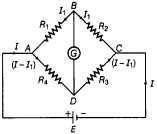On distributing currents as per Kirchhoff’s first rule.
Applying Kirchhoff’s second rule

1. In mesh ABDA,
{tex}\therefore{/tex} -I1 R1 + (I – I1) R4 = 0
{tex}\Rightarrow{/tex} I1 R1 = (I – I1) R4 ….(i)
2. In mesh BCDB,
-I1 R2 + (I – I1) R3 = 0
{tex}\Rightarrow{/tex} I1 R2 = (I – I1) R3 …(ii)
On dividing Eq. (i) by Eq. (ii), we get
{tex}\frac { I _ { 1 } R _ { 1 } } { I _ { 1 } R _ { 2 } } = \frac { \left( I – I _ { 1 } \right) R _ { 4 } } { \left( I – I _ { 1 } \right) R _ { 3 } }\Rightarrow\; \frac { R _ { 1 } } { R _ { 2 } } = \frac { R _ { 4 } } { R _ { 3 } }{/tex}
This is necessary and required balanced condition of balanced Wheatstone bridge.
1. Total resistance of parallel combination,
{tex}\frac{1}{R} = \frac{1}{{{R_1}}} + \frac{1}{{{R_2}}} + \frac{1}{{{R_3}}}{/tex} or {tex}\frac{1}{R} = \frac{1}{2} + \frac{1}{4} + \frac{1}{5} = \frac{{10 + 5 + 4}}{{20}} = \frac{{19}}{{20}}\Omega {/tex}
or {tex}R = \frac{{20}}{{19}}\Omega {/tex}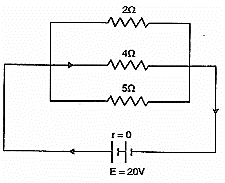2. Given voltage across the parallel
Combination V = 20 volt
Let the current through resistance {tex}2\Omega ,4\Omega {/tex} and {tex}5\Omega {/tex} are I1, I2 and I3 respectively.
Now {tex}{I_1} = \frac{V}{{{R_1}}} = \frac{{20}}{2} = 10A{/tex}
{tex}{I_2} = \frac{V}{{{R_2}}} = \frac{{20}}{4} = 5A{/tex}
{tex}{I_3} = \frac{V}{{{R_3}}} = \frac{{20}}{5} = 4A{/tex}
Total current, I = I1 + I2 + I3 = 10 + 5 + 4 = 19 A
7. For BCD, equivalent resistance
{tex}R _ { 1 } = 5 \Omega + 5 \Omega = 10 \Omega{/tex}
Across BA, equivalent resistance R2,
{tex}\frac { 1 } { R _ { 2 } } = \frac { 1 } { 10 } + \frac { 1 } { 30 } + \frac { 1 } { 15 }{/tex}
{tex}= \frac { 3 + 1 + 2 } { 30 } = \frac { 6 } { 30 } = \frac { 1 } { 5 }{/tex}
{tex}\Rightarrow \quad R _ { 2 } = 5 \Omega{/tex}
Potential difference between points A and B,
{tex}V _ { B A } = I \times R _ { 2 } = 0.2 \times 5{/tex}
{tex}V _ { B A } = 1 \mathrm { V } \Rightarrow V _ { A B } = – 1 \mathrm { V }{/tex}
8. In DC circuit, initially capacitor is in steady state i.e. it offers infinite resistance. Therefore, no current flows through capacitor and {tex}4\Omega{/tex} resistance, so resistance will produce no effect.
{tex}\therefore{/tex} In circuit AB the Effective resistance between {tex}2\Omega \ and\ 3 \Omega{/tex} which are connected in parallel combination is given by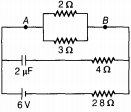{tex}R _ { A B } = \frac { 2 \times 3 } { 2 + 3 }{/tex}
{tex}= 1.2 \Omega \left[ \because \frac { 1 } { R_{eq} } = \frac { 1 } { R _ { 1 } } + \frac { 1 } { R _ { 2 } } \Rightarrow R = \frac { R _ { 1 } R _ { 2 } } { R _ { 1 } + R _ { 2 } } \right]{/tex}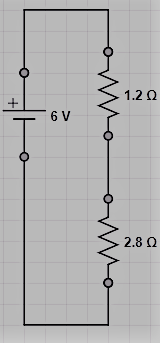Total resistance of the circuit = 1.2+ 2.8 = {tex}4\Omega{/tex} [{tex}\because{/tex} these two are connected in series combination] Net current drawn from the cell,
Given ,
Voltage =6v
total resistance(R){tex}=4\Omega{/tex}
{tex}I = \frac { V } { R ( \text { total resistance } ) } = \frac { 6 } { 4 } = \frac { 3 } { 2 } = 1.5 \mathrm { A }{/tex}
{tex}\therefore{/tex} Potential difference between A and B
{tex}V _ { A B } = I R _ { A B } = 1.5 \times 1.2 \Rightarrow V _ { A B } = 1.80 \mathrm { V }{/tex}
Current through {tex}2\Omega{/tex} resistance,
we know that V=I’R
{tex}I ^ { \prime } = V _ {AB} / 2 \Omega = 1.8 / 2 = 0.9 \mathrm { A }{/tex}
9. The resistance of the whole wire is {tex}8 \Omega{/tex} which is bent in the form of a circle.
When the wire is bent in circular form of radius ‘r’, the circumference of the circle is, L = 2πr
{tex}\Rightarrow r = {L\over2π}{/tex}
A and B are two opposite points on the wire when bent in circular form.
The resistance of each such part will be Resistance of one part between A and B is {tex}= {8\over L}[\pi r] = {8\over L}[\pi ({L\over 2\pi})]={8\over 2}= 4\Omega{/tex}
Similarly, resistance of the other part is also 4 Ω.
From the figure, it is clear that both the parts are in parallel combination.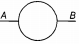So, effective resistance between A and B is given by,{tex}\frac { 1 } { R _ { A B } } = \frac { 1 } { R _ { 1 } } + \frac { 1 } { R _ { 2 } }{/tex}
{tex}\Rightarrow \quad \frac { 1 } { R _ { AB } } = \frac { 1 } { 4 } + \frac { 1 } { 4 }{/tex}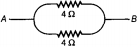{tex}\Rightarrow \quad R _ { A B } = \frac { 4 } { 1 + 1 } = 2 \Omega{/tex}

## Chapter Wise Extra Questions of Class 12 Physics Part I & Part II## Test Generator

Create Papers with your Name & Logo